Next: Four-Terminal Networks Up: Alternating Current Circuits Previous: Combined Impedances

# Resonance and the Transfer Function

Lets now consider putting a sinusoidal source in our series RCL circuit and consider the voltage across one of the circuit elements. The resistor for example in figure 2.7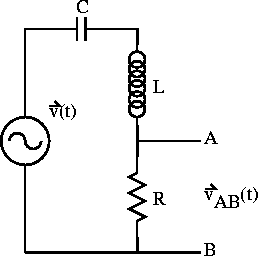Figure 2.7:  Driven series RCL circuit.

Applying Ohm's law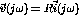across the resistor gives (cf. a voltage divider)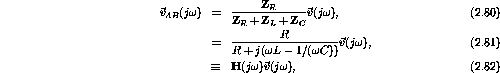where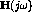is known as the transfer function in the frequency domain. We have changed independent variables fromtofor convenience.

We define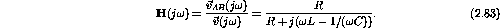contains all the information needed to characterize the circuit. In exponential form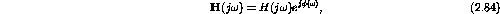where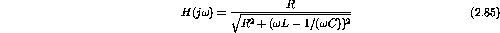andhas a maximum (resonance) given by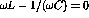. Or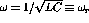is the resonant frequency.

Example: Consider the series LCR circuit (figure 2.8) driven by a voltage phasor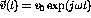.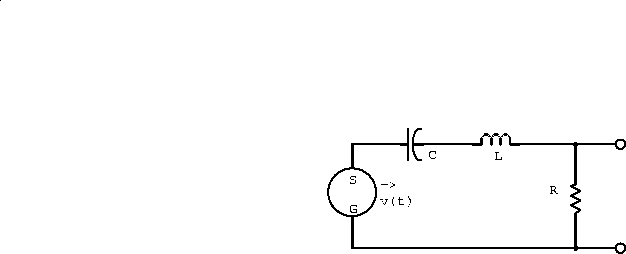Figure 2.8:  Driven series LCR circuit.

1. At an angular frequency such that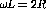and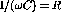, write the current phasor in terms of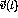and R.

v(t) is given by v(t)=Zi(t), where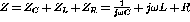. Atand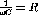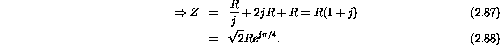Therefore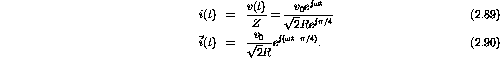2. At the instant whenis exactly real, calculate the three phasors representing the voltage developed across the R, C, and L circuit elements.

v(t) is real at t=0. Thus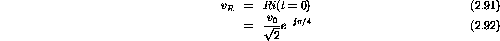And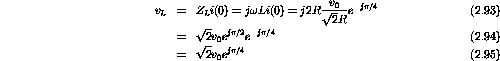Also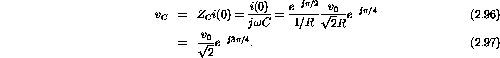3. Algebraically and with a sketch on the complex plane, show that the complex voltage sum around the closed loop is zero.

The three voltage phasors are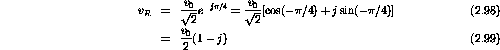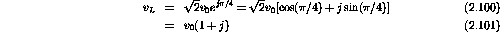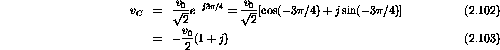Around the closed loop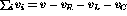. If this expression is zero at t=0 it will be zero for all time. Therefore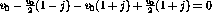.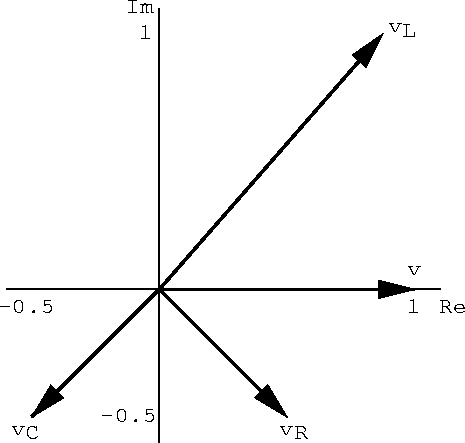Figure 2.9: Complex voltage sum around the closed loop of the driven LCR circuit.

Example: Sketch simplified versions of the circuit shown in figure 2.10 that would be valid at: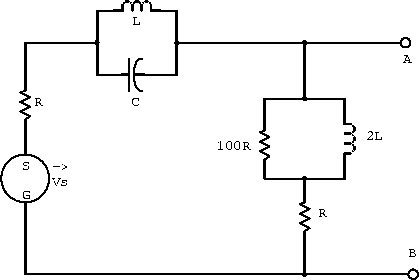Figure 2.10:  Example LCR circuit.

1.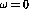;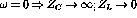.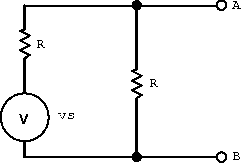Figure 2.14: Example circuit for.

2. very low frequencies but not;

Whenis small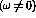C and L are in parallel and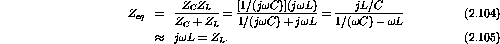2L and 100R in parallel gives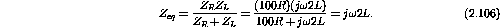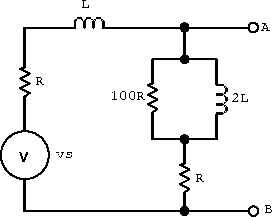Figure 2.12: Example circuit for very low frequencies but not.

3. very high frequencies but not;large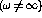(note: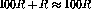).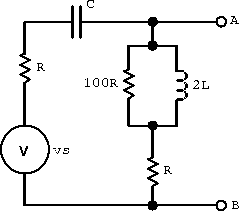Figure 2.13: Example circuit for very high frequencies but not.

4..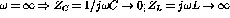.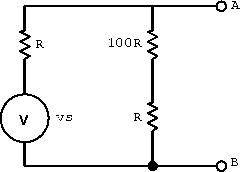Figure: Example circuit for.

Example: For the circuit shown in figure 2.15 plot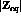as a function of frequency over the range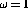rad/s to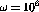rad/s.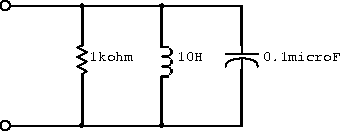Figure 2.15:  Example circuit with components in parallel.

The equivalent impedance for the three components in parallel is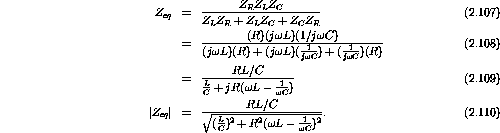Plugging in the numerical values gives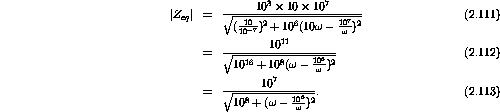A table of values and its plot follows.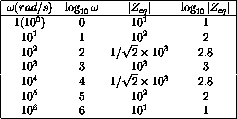Table 2.1: Numerical values for example circuit.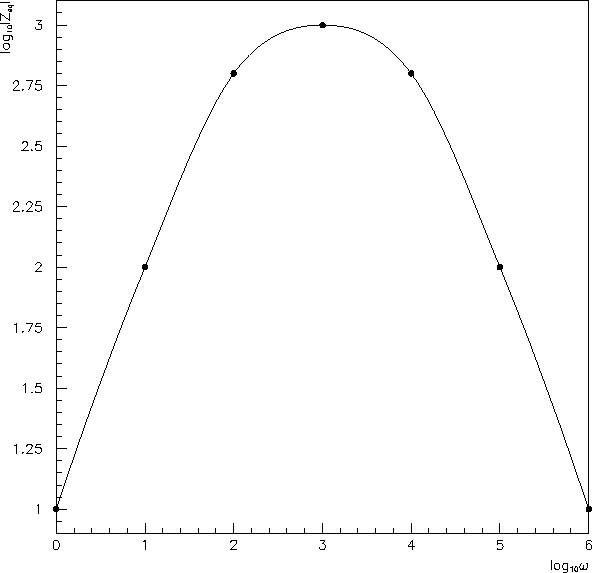Figure 2.16: Plot of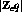for example circuit.Next: Four-Terminal Networks Up: Alternating Current Circuits Previous: Combined Impedances

Doug Gingrich
Tue Jul 13 16:55:15 EDT 1999